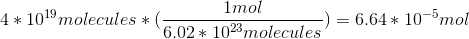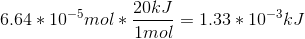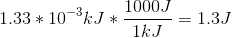# MCAT Physical : Energetics of Biological Reactions and ATP

## Example Questions

### Example Question #1 : Thermochemistry And Energetics

Adenosine-triphosphate is formed by the following endothermic reaction.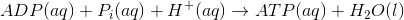If body temperature decreases, ADP production __________.

remains constant

decreases

increases

is unpredictable

increases

Explanation:

Since heat can be considered a reactant (endothermic), if heat is decreased, ADP is increased according to Le Chatelier’s Principle. If a reactant is removed, the equilibrium will shift toward the reactants. In this reaction, ADP and heat are both reactants.

### Example Question #1 : Thermochemistry And Energetics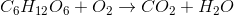Which of the following is true, assuming the reaction goes to completion?

I. There is an increase in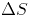, entropy

II.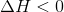III. At equilibrium,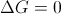IV. Oxygen is the reducing agent

II and III

II only

I and III

I, II, and III

I and IV

I, II, and III

Explanation:

This question ties together the reaction for oxidation of glucose with several thermodynamic principles.

The first option, stating that there is an increase of entropy, is true. When balanced, we see that there is an increase in total number of molecules when the reaction completes; thus, there will be an increase in entropy.

The second option states that enthalpy, or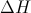, is less than than zero. This is also true, as it implies that this is an exothermic (or heat generating) reaction. The oxidation of glucose is how our bodies generate heat and keep homeostasis.

The third option, stating that Gibb's free energy is zero at equilibrium, is also true. Any equation will haveat equilibrium.

The last option, stating that oxygen is the reducing agent, is false. Oxygen is actually the oxidizing agent and is itself reduced.

### Example Question #1 : Energetics Of Biological Reactions And Atp

ATP stores energy through its ability to lose a phosphate group to form ADP in the following reaction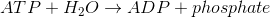At body temperature, the thermodynamic values for ATP hydrolysis are as follow.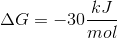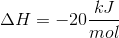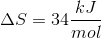Glycolysis is an energy-liberating process (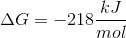,) that is coupled with the conversion of two ADP molecules into two ATP molecules according to the following reaction.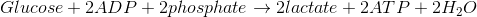Glycolysis results in which of the following?

A conservation of entropy

A decrease in entropy

An increase in entropy

An increase in enthalpy

An increase in entropy

Explanation:

Since glycolysis is an exothermic reaction (ΔH is negative), enthalpy does not increase. As for entropy, the reaction begins with a large molecule and ends with smaller molecules, therefore entropy increases.

### Example Question #4 : Thermochemistry And Energetics

ATP stores energy through its ability to lose a phosphate group to form ADP in the following reactionAt body temperature, the thermodynamic values for ATP hydrolysis are as follow.
ΔG = –30kJ/mol
ΔH = –20kJ/mol
ΔS = 34kJ/mol

Glycolysis is an energy-liberating process (ΔG = –218kJ/mol, ΔH = –20 kJ/mol) that is coupled with the conversion of two ADP molecules into two ATP molecules according to the following reaction.Glycolysis is __________.

exergonic and exothermic

exergonic and endothermic

endergonic and endothermic

endergonic and exothermic

exergonic and exothermic

Explanation:

ΔG and ΔH are both negative for glycolysis, meaning that the reaction is exergonic and exothermic.

### Example Question #5 : Thermochemistry And Energetics

ATP stores energy through its ability to lose a phosphate group to form ADP in the following reactionAt body temperature, the thermodynamic values for ATP hydrolysis are as follow.
ΔG = –30kJ/mol
ΔH = –20kJ/mol
ΔS = 34kJ/mol

Glycolysis is an energy-liberating process (ΔG = –218kJ/mol, ΔH = –20 kJ/mol) that is coupled with the conversion of two ADP molecules into two ATP molecules according to the following reaction.The formation of peptide bonds requires 17kJ/mol of energy. It also requires the energy of three ATP molecules. How many ATP molecules are required to synthesize a protein containing 550 peptide bonds?

550

1,962

1,650

312

1,962

Explanation:

Since we know that three ATP molecules per bond are required, 1,650 ATP molecules are needed. However, we also know that 17kJ/mol of additional energy is required for each bond. This energy must come from ATP molecules. The only choice that is greater than 1,650 is 1,962.

### Example Question #6 : Thermochemistry And Energetics

ATP stores energy through its ability to lose a phosphate group to form ADP in the following reactionAt body temperature, the thermodynamic values for ATP hydrolysis are as follow.
ΔG = –30kJ/mol
ΔH = –20kJ/mol
ΔS = 34kJ/mol

Glycolysis is an energy-liberating process (ΔG = –218kJ/mol, ΔH = –20 kJ/mol) that is coupled with the conversion of two ADP molecules into two ATP molecules according to the following reaction.How much heat, in joules, is released when 4 * 1019 molecules of ATP are converted to ADP via ATP hydrolysis?

45J

13,000J

1.3 * 10-3J

1.3J

1.3J

Explanation:

To solve this problem, first convert molecules to moles with Avogadro’s number, then convert moles to kJ using the ΔH of ATP hydrolysis. Lastly convert kJ to J.